# Summary of Chapter 8: Potential Energy and Conseration of Energy

Conservative forces The net work of conservative force moving a particle around on a closed path, from an initial point and back is zero. Similarly, the path taken between two points while moving an object does not affect the work done on the object. Gravity and any spring force are conservative. Friction is a nonconservative force.

Potential Energy Potential energy is dependent on the arrangement of a system. the change in potential energy is equal to the work done by conservative forces in a system: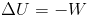If the particle moves from xi to xf, the change in potential energy is given by: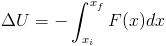Gravitational Potential Energy: The potential energy due to gravity is given by: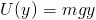where m is the particle's mass, g is 9.8 m/s2 and y is the height of the particle in meters. The height is measured from a constant refrence point chosen as y=0 meters

Elastic Potential Energy Elastic potential energy is energy stored in a compressed or stretched spring. The value of this energy is given by: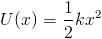Where k is a spring constant and x is a distance also measured from a refrence point, however, the refrence point of x=0 is usually set to the relaxed state of the spring.

Mechanical Energy Mechanical energy is the sum of the kinetic energy and potential energy of the system: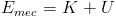An isolated system is one in which no externam forces cause energy changes. If this is true, then the mechanical energy of a system cannot change. This represents the principal of conservation of energy: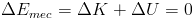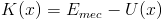Work by External Forces Work is energy transferd to or from a system by external forces. Therefore, work changes mechanical energy:When frictional forces act within a system (these are non conservative forces), they remove energy in the form of thermal energy. This is then represented by: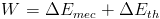The change in thermal energy is related to the magnetude of the frictional force (fk) and the distance over which it acts (d)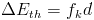Conservation of Energy The total energy of a system can change only change by amounts transered to and from the system. Energy cannot be created or destoryed anywhere in the universe.

Power The power due to a force is the rate at which that force transers energy. Therefore power is a change in energy over a change in time: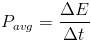Return to top
All information and equations are taken from and credited to Jearl Walker's Fundamental Physics.